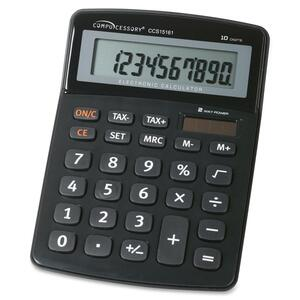Calculators\$CALL

SKU: CCS15161

10-Digit Handheld Calculator

Handheld calculator features a large, 10-digit display with a tilt head so you can easily see your calculations. Functions include clear entry, memory add, memory subtract, memory clear, square foot, percent, tax, add, subtract, multiply, divide, total/equal key, and plus/minus key. Calculator runs on solar and battery power.

Simple Calculator
Compucessory
Battery, Solar

• Clear entry
• Memory subtract
• Memory clear
• Square foot
• Percentage
• Tax
• Subtract
• Multiply
• Division
• Total/Equal
• Plus/Minus
China
0.3″
4.1″
5.5″
White
Compucessory
10-Digit Handheld Calculator
10-digit
15161
http://www.compucessory.com